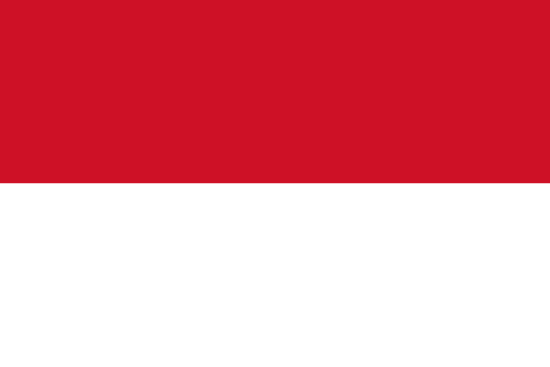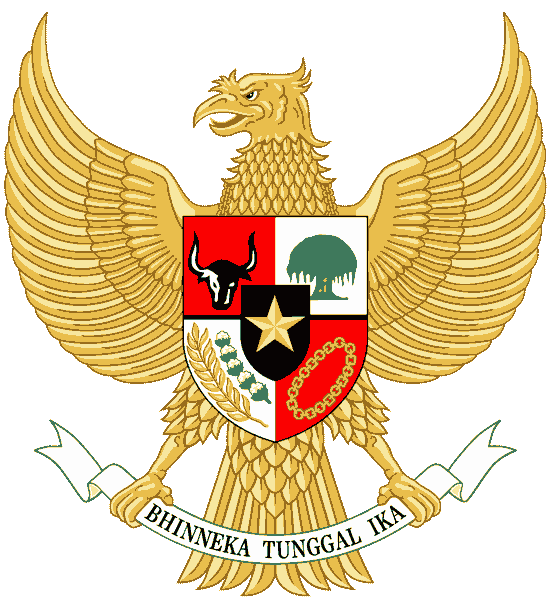印尼语字母及发音表 大写 小写 发音 大写 小写 发音 A a a N n en B b be O o o C c ce P p pe D d de Q q ki E e e R r er F f ef S s es G g ge T t te H h ha U u u I i i V v fe J j je W w we K k ka X x eks L l el Y y ye M m em Z z zet